# Knowledge Base

## Solution 34537: Attaching a Formula to a List Name in Stat List Editor Using the TI-84 Plus C Silver Edition Graphing Calculator.

### How can I attach a formula to a list name in Stat List Editor using the TI-84 Plus C Silver Edition graphing calculator?

A formula can be attached to a list name in the stat list editor and then display and edit the calculated list elements. When executed the attached formula must resolve to a list.

To attach a formula to a list name that is stored in the stat list editor, follow the steps below using the example of L1={1,2,3} and L2={2,3,4}.

• Press [STAT] .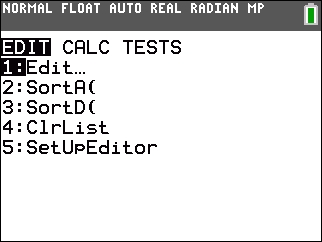• Press  [ENTER]  [ENTER]  [ENTER].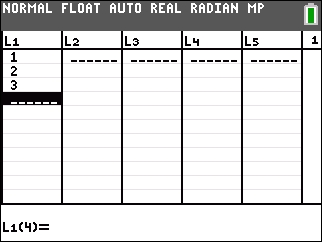• Press [►]  [ENTER]  [ENTER]  [ENTER].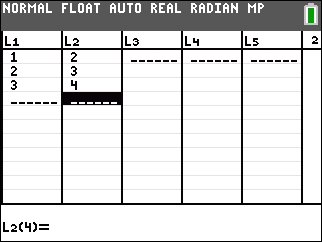• Press [►] [▲] [ENTER].
• Press [ALPHA] [].
• Press [2ND] [L1] [+] [2ND] [L2].
• Press [ALPHA] [“] [ENTER].

NOTE: If a formula in quotation marks is displayed on the entry line, then a formula is already attached to the list name. To edit the formula, press [ENTER], and then edit the formula.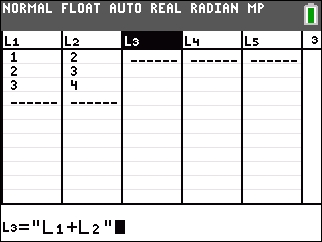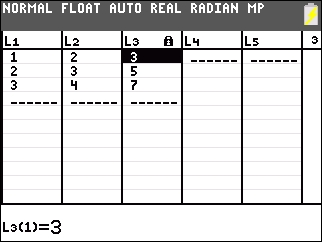Please Note: If quotation marks are not used the handheld calculates and displays the same initial list of answers, but does not attach the formula for future calculations.

Please see the TI-84 Plus C Silver Edition guidebook for additional information.